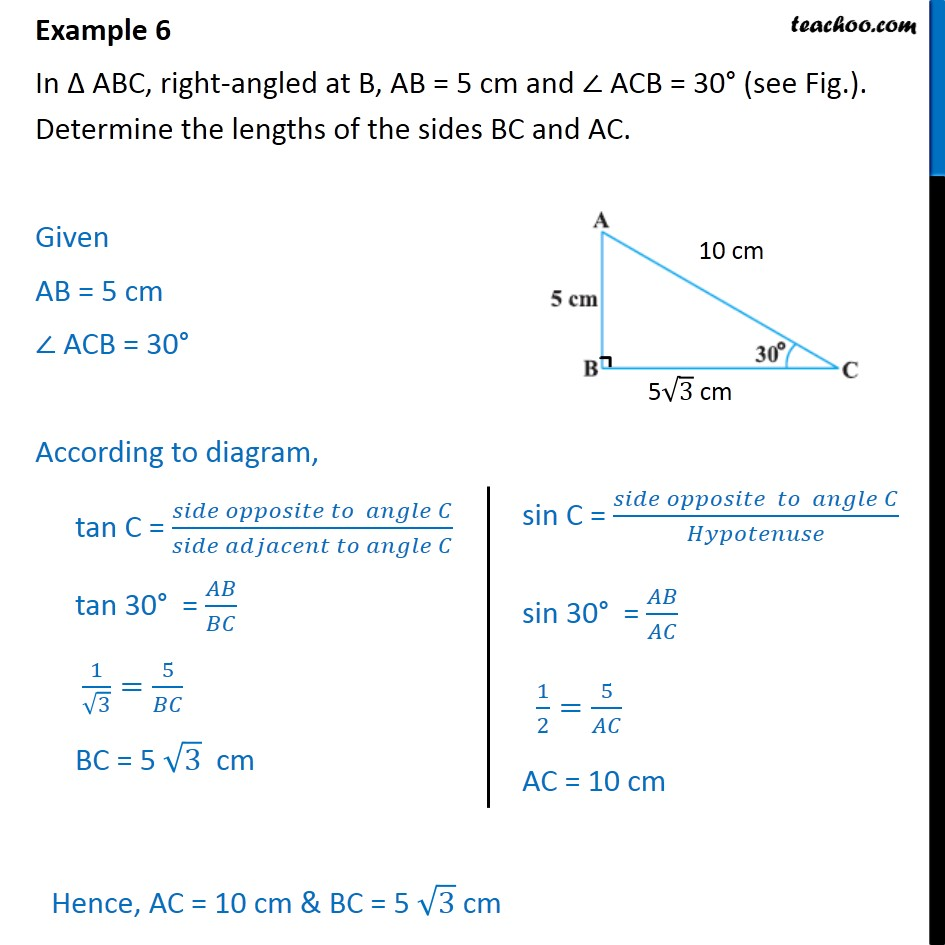1. Chapter 8 Class 10 Introduction to Trignometry (Term 1)
2. Serial order wise
3. Examples

Transcript

Example 6 In Δ ABC, right-angled at B, AB = 5 cm and ∠ ACB = 30° (see Fig.). Determine the lengths of the sides BC and AC. Given AB = 5 cm ∠ ACB = 30° According to diagram, tan C = (𝑠𝑖𝑑𝑒 𝑜𝑝𝑝𝑜𝑠𝑖𝑡𝑒 𝑡𝑜 𝑎𝑛𝑔𝑙𝑒 𝐶)/(𝑠𝑖𝑑𝑒 𝑎𝑑𝑗𝑎𝑐𝑒𝑛𝑡 𝑡𝑜 𝑎𝑛𝑔𝑙𝑒 𝐶) tan 30° = 𝐴𝐵/𝐵𝐶 1/√3=5/𝐵𝐶 BC = 5 √3 cm

Examples

Chapter 8 Class 10 Introduction to Trignometry (Term 1)
Serial order wise